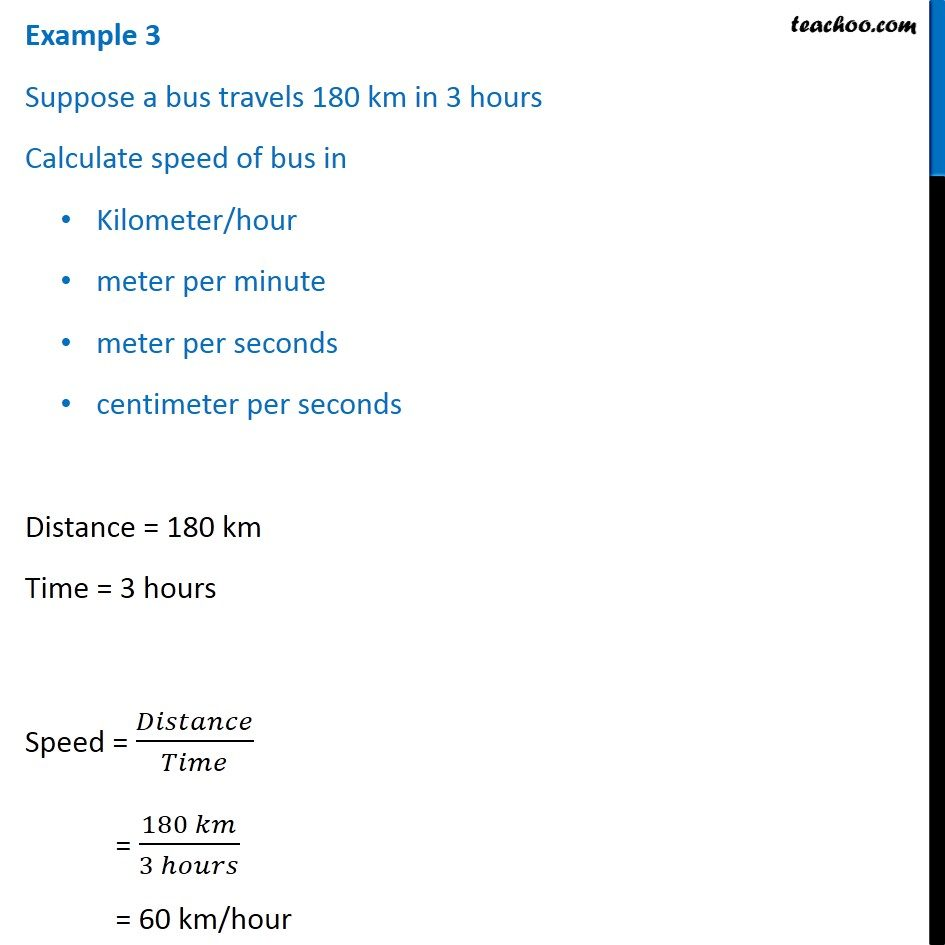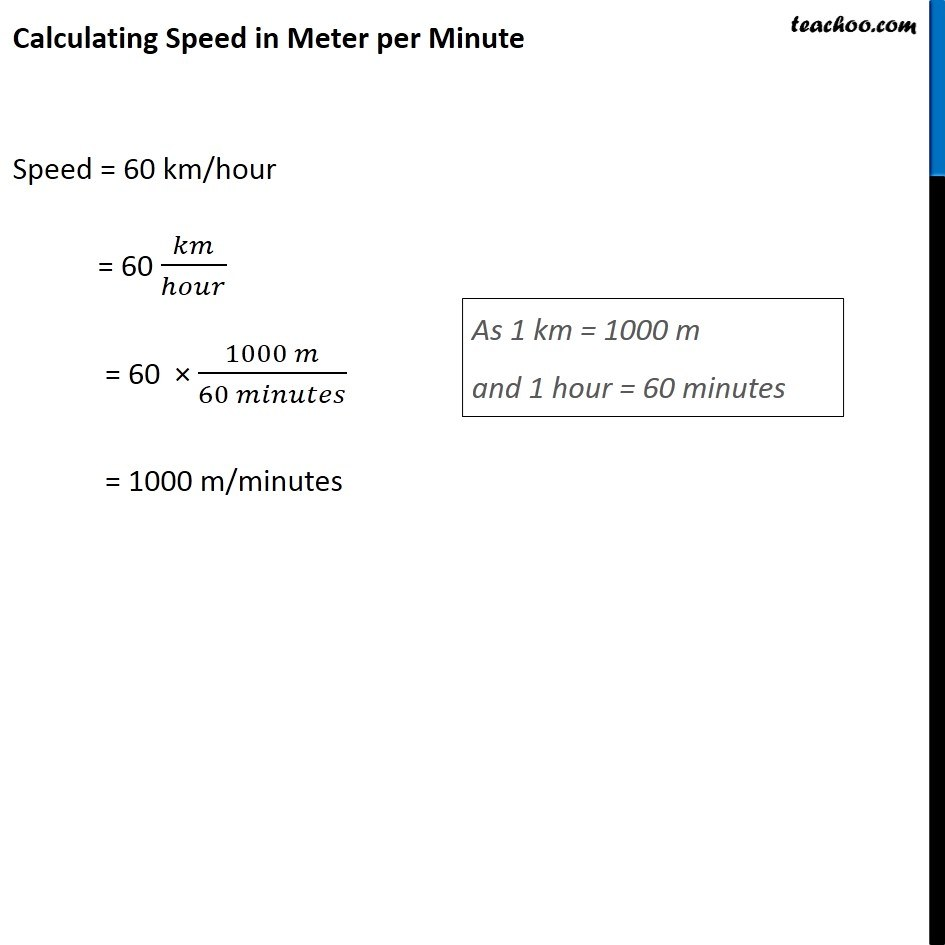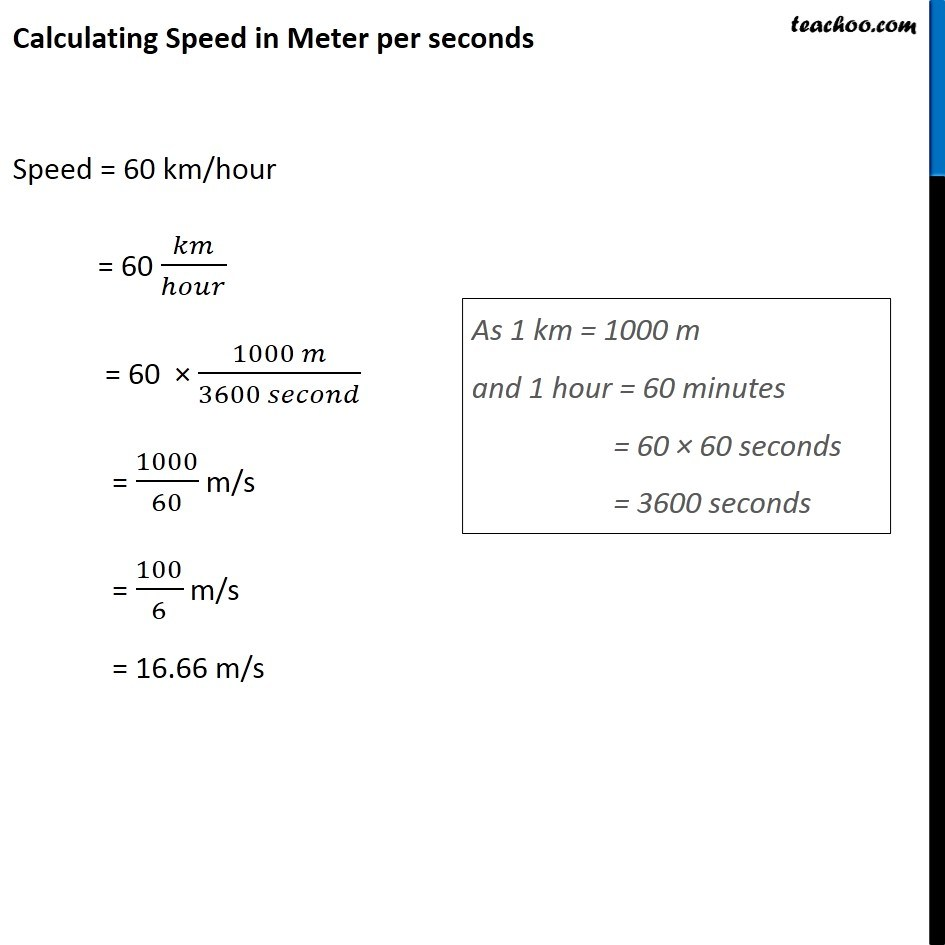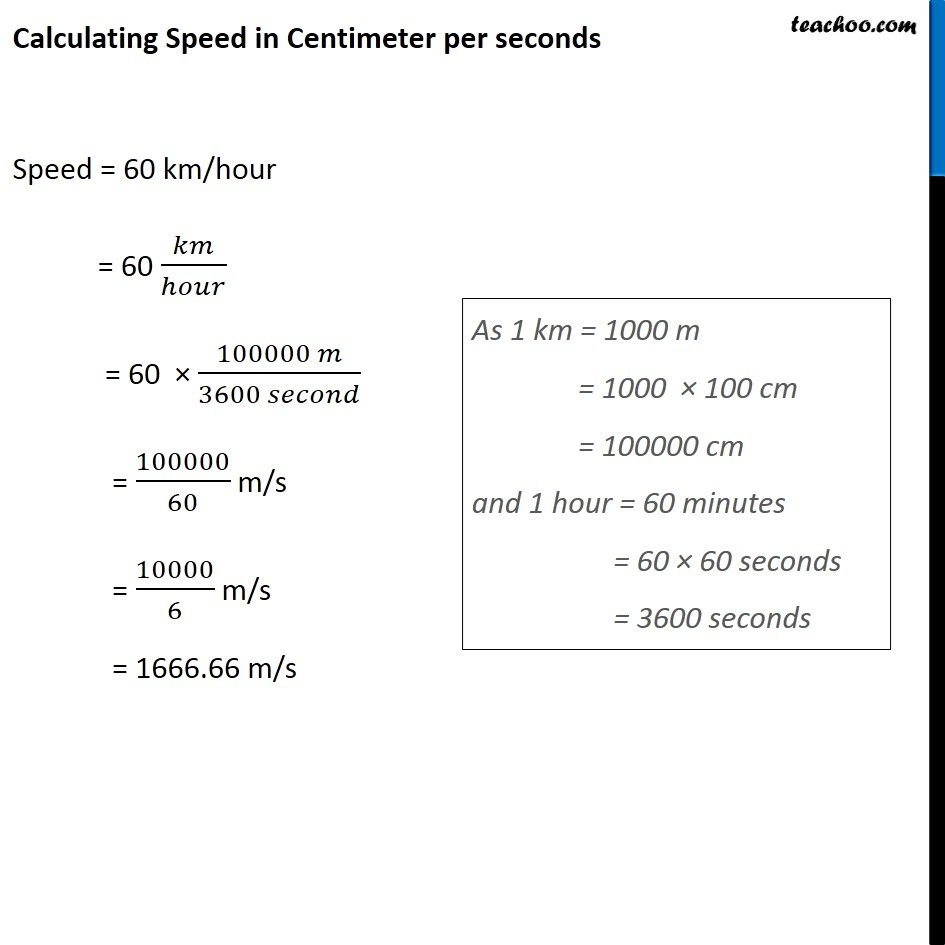Concepts

Class 9
Chapter 8 Class 9 - Motion

Suppose a person travels distance of 6 kilometers in 2 hours

What is his speed

His Speed is 3 Kilometers per hour

## What is Speed

It is distance travelled per unit of time

#### How to Calculate Speed

Speed = Distance/Time

How is it measured

It is normally measured in kilometer per hour

Shorter Distances are also measured in meter per seconds

## Example 1

Suppose an aeroplane travels distance of 1600 kilometers in 2 Hours

What is speed of Plane

Distance = 1600 km

Time = 2 hours

Speed = Distance/Time

= 1600/2

= 800 Km/Hour

## Example 2

Suppose an athlete runs 200 meter race in 25 seconds

What is his speed in meter per second?

Distance = 200 m

Time = 25 Seconds

Speed = Distance/Time

Speed = 200 /25 = 8 meter per second

Note

1 Km = 1000 meters

I Meter = 100 centimeters

I hour = 60 Minutes

1 Minutes = 60 Seconds

Now, lets find speed in different units## Questions

Q 3 Page 102 - What does the odometer of an automobile measure?

Q 5 Page 102 - A driver of a car travelling at 52 km h–1 applies the brakes and accelerates uniformly in the opposite direction. The car stops in 5 s. Another driver going at 3 km h–1 in another car applies his brakes slowly and stops in 10 s. On the same graph paper, plot the speed versus time graphs for the two cars. Which of the two cars travelled farther after the brakes were applied?

Learn in your speed, with individual attention - Teachoo Maths 1-on-1 Class

### Transcript

Example 3 Suppose a bus travels 180 km in 3 hours Calculate speed of bus in Kilometer/hour meter per minute meter per seconds centimeter per seconds Distance = 180 km Time = 3 hours Speed = 𝐷𝑖𝑠𝑡𝑎𝑛𝑐𝑒/𝑇𝑖𝑚𝑒 = (180 𝑘𝑚)/(3 ℎ𝑜𝑢𝑟𝑠) = 60 km/hour Calculating Speed in Meter per Minute Speed = 60 km/hour = 60 𝑘𝑚/ℎ𝑜𝑢𝑟 = 60 × (1000 𝑚)/(60 𝑚𝑖𝑛𝑢𝑡𝑒𝑠) = 1000 m/minutes As 1 km = 1000 m and 1 hour = 60 minutes Calculating Speed in Meter per seconds Speed = 60 km/hour = 60 𝑘𝑚/ℎ𝑜𝑢𝑟 = 60 × (1000 𝑚)/(3600 𝑠𝑒𝑐𝑜𝑛𝑑) = 1000/60 m/s = 100/6 m/s = 16.66 m/s As 1 km = 1000 m and 1 hour = 60 minutes = 60 × 60 seconds = 3600 seconds Calculating Speed in Centimeter per seconds Speed = 60 km/hour = 60 𝑘𝑚/ℎ𝑜𝑢𝑟 = 60 × (100000 𝑚)/(3600 𝑠𝑒𝑐𝑜𝑛𝑑) = 100000/60 m/s = 10000/6 m/s = 1666.66 m/s As 1 km = 1000 m = 1000 × 100 cm = 100000 cm and 1 hour = 60 minutes = 60 × 60 seconds = 3600 seconds